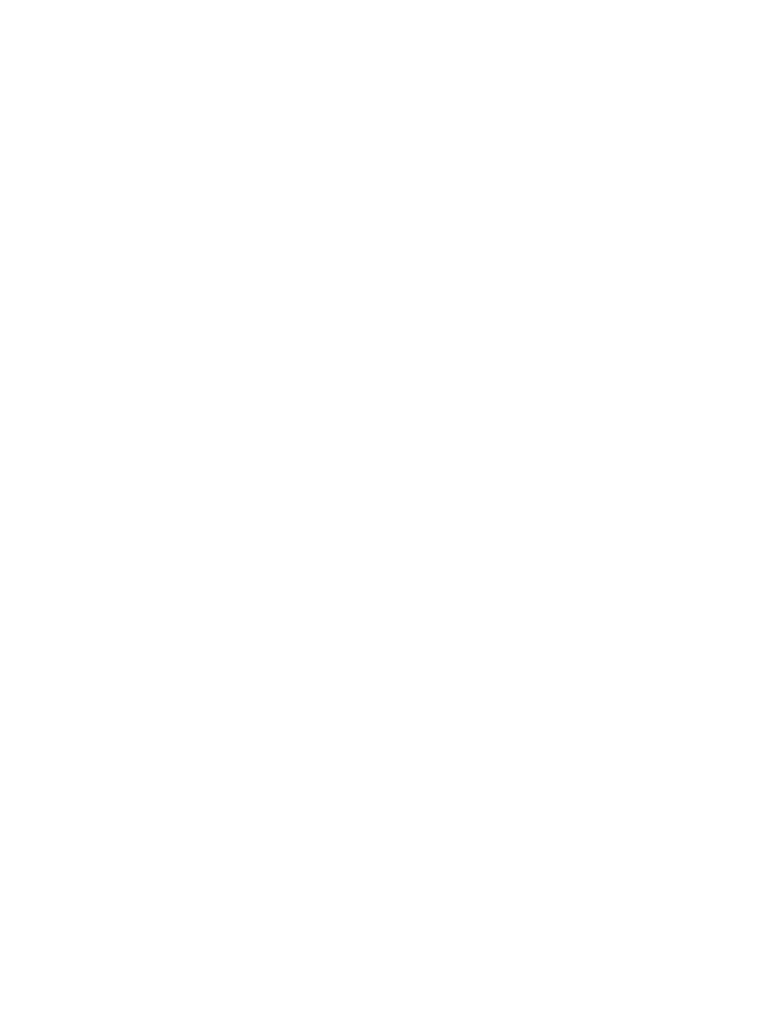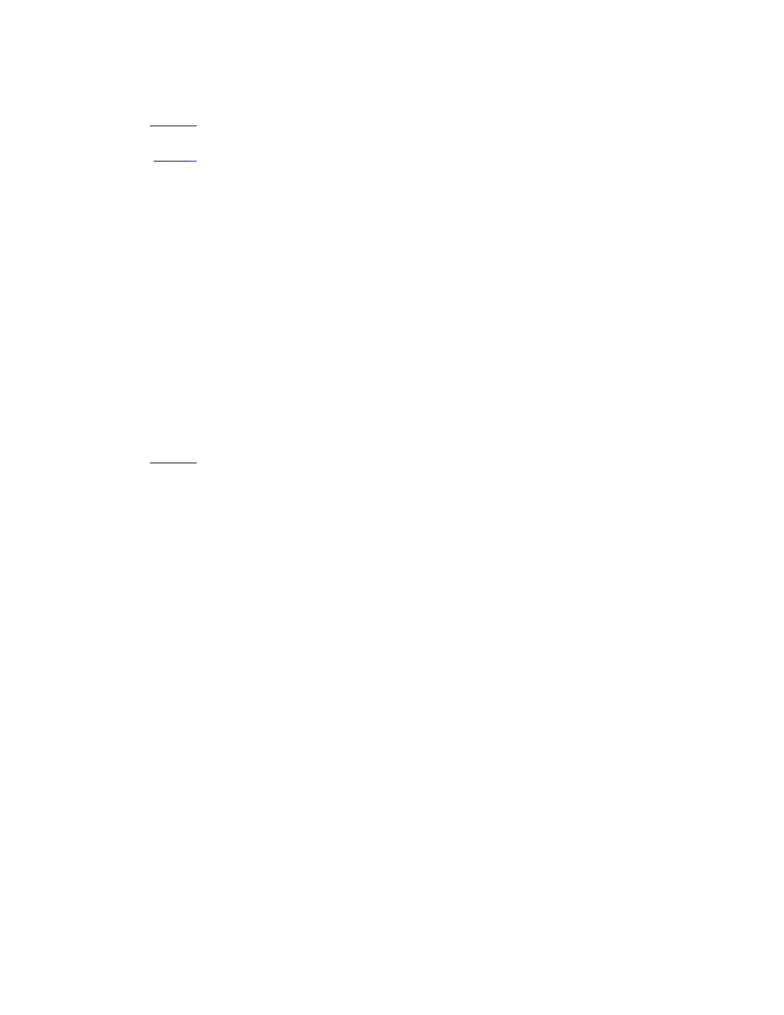Textbook Notes (270,000)
CA (160,000)
York (10,000)
ITEC (100)
Chapter

Department
Information Technology
Course Code
ITEC 1000
Professor
Peter Khaiter

This preview shows pages 1-2. to view the full 7 pages of the document.AP/ITEC1000 Sections A and B
"Introduction to Information Technologies"
Fall 2011
Assignment # 2
1)
a) Convert 121 to its binary representation: 12110 = 011110012. Positive numbers are always
represented by themselves.
b) Convert -121 to its binary representation: -12110 = -011110012. Using the second method
for negative numbers, invert and then add 1.
c) Convert 54 to its binary representation: 5410 = 001101102. Positive numbers are always
represented by themselves.
2) Yes. The four least significant bits of the EBCDIC code match the four least significant digits
of ASCII.
3) Between -79999 and +79999. This is because 20 bits provide five four-bit locations. The
lowest (right most) and three middle 4 bit groups can each store any number from 0 to 9. In the
highest (left most) 4 bits, one bit is used for the sign, leaving 3 bits for digits. These 3 bits can
accommodate numbers between 0 and 7.
4)
a) 15765 is a positive number and is represented by itself
99999 - 8773 = 91226

Only pages 1-2 are available for preview. Some parts have been intentionally blurred.b) 15765
+91226
106991 add the carry into the result
+ 1
06992
The leading digit in the complement is 0. Therefore, it represents a positive decimal
number: 6992.
c) There was no overflow condition because the inputs in the sum have opposite signs and
overflow cannot occur.
There was a carry condition because in the result, the number of digit exceeded the
specified number (i.e., five) and the carry was added to the result.
d) 15765 is a positive number and is represented by itself
100000 - 8773 = 91227
e) 15765
+91227
106992 Ignore the carry and the result is: 6992
bit to the result.
Radix complement arithmetic does not require this step, because the carry is simply
ignored. The latter is easier.
5) Replace each decimal digit by its 4-bit BCD representation and add the corresponding sign
pattern in the low-order bits.
a) -924 = 1001001001001101 b) 80356 = 100000000011010101101111 c)
+981 = 1001100000011100
6)
a) The leading digit is between 0 and 4 and, therefore, represents a positive number. Positive
numbers are represented by themselves.